# NCERT Solutions for Class 10 Maths Chapter 12 Areas Related to Circles Ex 12.2

Get Free NCERT Solutions for Class 10 Maths Chapter 12 Ex 12.2 PDF. Areas Related to Circles Class 10 Maths NCERT Solutions are extremely helpful while doing your homework. Exercise 12.2 Class 10 Maths NCERT Solutions were prepared by Experienced ncert-books.in Teachers. Detailed answers of all the questions in Chapter 12 Maths Class 10 Areas Related to Circles Exercise 12.2 provided in NCERT TextBook.NCERT Solutions for Class 10 Maths Chapter 12 Areas Related to Circles Ex 12.2

NCERT Solutions for Class 10 Maths Chapter 12 Areas Related to Circles Ex 12.2 are part of NCERT Solutions for Class 10 Maths. Here we have given NCERT Solutions for Class 10 Maths Chapter 12 Areas Related to Circles Ex 12.2

Question 1.
Find the area of a sector of a circle with radius 6 cm if angle of the sector is 60°.
Solution: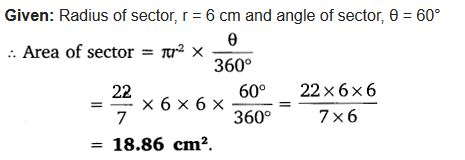Question 2.
Find the area of a quadrant of a circle whose circumference is 22 cm.
Solution: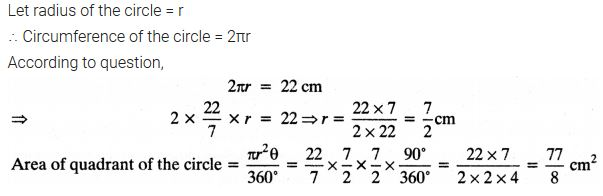Question 3.
The length of the minute hand of a clock is 14 cm. Find the area swept by the minute hand in 5 minutes.
Solution: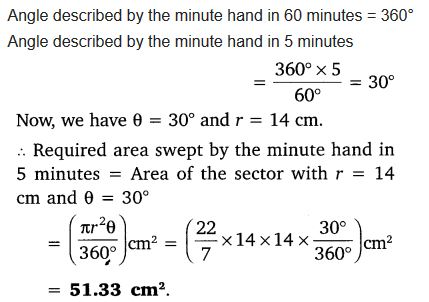Question 4.
A chord of a circle of radius 10 cm subtends a right angle at the centre. Find the area of the corresponding:
(i) minor segment
(ii) major segment (Use π = 3.14)
Solution:Question 5.
In a circle of radius 21 cm, an arc subtends an angle of 60° at the centre. Find:
(i) length of the arc.
(ii) area of the sector formed by the arc.
(iii) area of the segment formed by the corresponding chord.
Solution:Question 6.
A chord of a circle of radius 15 cm subtends an angle of 60° at the centre. Find the areas of the corresponding minor and major segments of the circle. (Use π = 3.14 and √3 = 1.73)
Solution:Question 7.
A chord of a circle of the radius 12 cm subtends an angle of 120° at the centre. Find the area of the corresponding segment of the circle. (Use π = 3.14 and √3 = 1.73).
Solution:Question 8.
A horse is tied to a peg at one corner of a square shaped grass field of side 15 m by means of a 5 m long rope (see figure). Find:(i) the area of that part of the field in which the horse can graze.
(ii) the increase in the grazing area if the rope were 10 m long instead of 5 m. (Use π = 3.14)
Solution:Question 9.
A brooch is made with silver wire in the form of a circle with diameter 35 mm. The wire is also used in making 5 diameters which divide the circle into 10 equal sectors as shown in figure.
Find:(i) the total length of the silver wire required.
(ii) the area of each sector of the brooch.
Solution: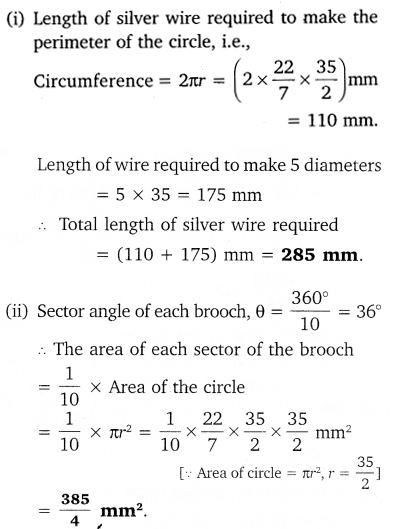Question 10.
An umbrella has 8 ribs which are equally spaced (see figure). Assuming umbrella to be a flat circle of radius / 45 cm, find the area between the two consecutive ribs of the umbrella.Solution:Question 11.
A car has two wipers which do not overlap.
Each wiper has a blade of length 25 cm sweeping through an angle of 115°. Find the total area cleaned at each sweep of the blades.
Solution:Question 12.
To warn ships for underwater rocks, a lighthouse spreads a red colored light over a sector of angle 80° to a distance of 16.5 km. Find the area of the sea over which the ships are warned. (Use π = 3.14)
Solution: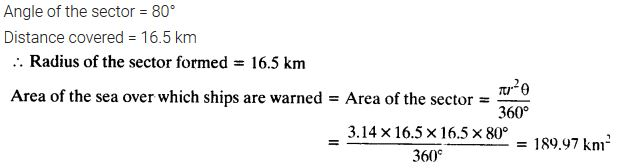Question 13.
A round table cover has six equal designs as shown in the figure. If the radius of the cover is 28 cm, find the cost of making the designs at the rate of ₹0.35 per cm². (Use √3= 1.7)Solution: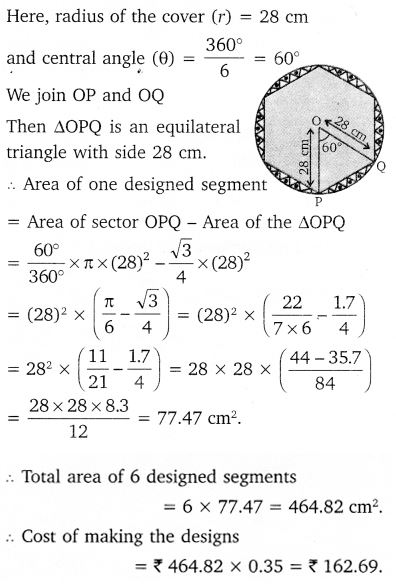Question 14.
Tick the correct answer in the following: Area of a sector of angle p (in degrees) of a circle with radius R isSolution:error: Content is protected !!
+